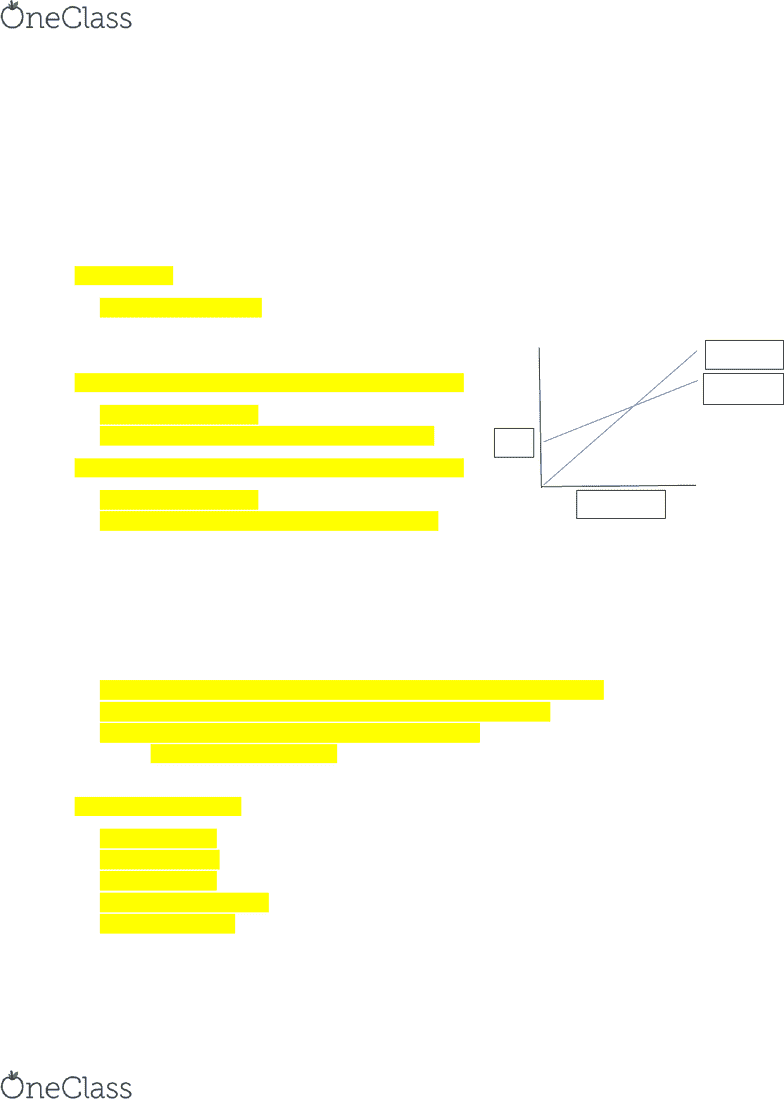Study Guides (400,000)
CA (150,000)
York (10,000)
ECON (900)
ECON 1010 (200)
Study Guide

# ECON 1010- Midterm Exam Guide - Comprehensive Notes for the exam ( 28 pages long!)

Department
Economics
Course Code
ECON 1010
Professor
Steven Edwards
Study Guide
Midterm

This preview shows pages 1-3. to view the full 28 pages of the document.York
ECON 1010
MIDTERM EXAM
STUDY GUIDE

Only pages 1-3 are available for preview. Some parts have been intentionally blurred.Only pages 1-3 are available for preview. Some parts have been intentionally blurred.Ch. 27 Expenditure Multipliers
Recall: Two ways to calculate Real GDP:
Income approach Aggregate income = real GDP
Expenditure approach Aggregate expenditure = real GDP
Aggregate expenditure
Y = C + I + G + (X-M)
Only C and M (consumption and imports) are influenced by real GDP
Disposable income (YD) = aggregate income/real GDP (taxes + transfer payments) = aggregate
income/real GDP net taxes
Therefore, YD = Y T
Disposable income is either consumed or spent
Therefore, YD = C + S
Consumption function the relationship between consumption expenditure and disposable income
Marginal propensity to consume (MPC) = C/YD
o It is the fraction of a change in disposable income spent on consumption
Saving function the relationship between saving and disposable income
Marginal propensity to save (MPS) = ∆S/∆YD
o It is the fraction of a change in disposable income that is saved
MPC + MPS always equal 1
C + S = YD
S + C = YD
C/∆YD + ∆S/∆YD = YD/∆YD
MPC + MPS = 1
Recall: Disposable income = aggregate income/real GDP taxes YD = Y - T
Thus, disposable income changes when real GDP or taxes change
When ta rates don’t change, the onl influence on disposable income is aggregate income/real
GDP
Therefore ∆YD = ∆Y
o MPC = C/Y
C = MPC*Y
o MPS = S/Y
Import function relationship between real GDP and imports
Marginal propensity to import (MPI) = ∆M/∆Y
find more resources at oneclass.com
find more resources at oneclass.com

Unlock to view full version

Only pages 1-3 are available for preview. Some parts have been intentionally blurred.Induced expenditure = C M (changes when real GDP changes)
Autonomous expenditure = I + G + X (does not change when real GDP changes)
Amount of consumption expenditure when disposable income = 0
Changes in autonomous expenditure shift the aggregate expenditure curve by the amount of
the change in autonomous expenditure
Aggregate expenditure = Induced expenditure + Autonomous expenditure
If AE = real GDP
Expenditure is in eqm
No unplanned changes in business inventories no changes in prod no changes in real GDP
Everone’s spending plans are fulfilled
If AE > real GDP (and AE and real GDP < eqm AE and real GDP)
i.e. AE above 45 line
Inventories dec inc production inc real GDP
If AE < real GDP (and AE and real GDP > eqm AE and real GDP)
i.e. AE below 45 line
Inventories inc dec production dec real GDP
When autonomous expenditure (when disposable income = 0) changes, so does eqm expenditure, but
the change in eqm expenditure is much larger than the change in autonomous expenditure
The factor by which it is larger is called the multiplier
The multiplier amount by which a change in autonomous expenditure is multiplied to determine the
change in eqm expenditure and real GDP
Multiplier = change in eqm expenditure/change in autonomous expenditure
Multiplier = change in real GDP/change in autonomous expenditure
Multiplier = 1/MPS (when there are no taxes or imports)
o Or Multiplier = 1/(1-MPC)
Change in real GDP = multiplier * change in autonomous expenditure
Multiplier increases when:
MPC increases
MPS decreases
MPI decreases
Income taxes decrease
Imports decrease
Real GDP
AE
AE Curve
45 line
find more resources at oneclass.com
find more resources at oneclass.com